Submit a blog to KDnuggets -- Top Blogs Win A Reward

Topics: AI | Data Science | Data Visualization | Deep Learning | Machine Learning | NLP | Python | R | Statistics

KDnuggets Home » News » 2018 » Feb » Tutorials, Overviews » Recommender Engine - Under The Hood ( 18:n09 )

# Recommender Engine - Under The Hood

We examine two main types of recommender systems: Content based and Collaborative filtering. Both have their pros and cons depending upon the context in which you want to use them.commentsMany of us are bombarded with various recommendations in our day to day life, be it on e-commerce sites or social media sites. Some of the recommendations look relevant but some create range of emotions in people, varying from confusion to anger.

There are basically two types of recommender systems, Content based and Collaborative filtering. Both have their pros and cons depending upon the context in which you want to use them.

Content based: In content based recommender systems, keywords or properties of the items are taken into consideration while recommending an item to an user. So, in a nutshell it is like recommending similar items. Imagine you are reading a book on data visualization and want to look for other books on the same topic. In this scenario, content based recommender system would be apt.Collaborative Filtering: Well to drive home the point, the below picture is the best example. Customer A has bought books x,y,z and customer B has bought books y,z. Now collaborative filtering technique would recommend book x to customer B. This is both the advantage and disadvantage of collaborative filtering. It does not matter if the book x was a nonfiction book while the liking of customer B was strictly fiction book. The relevancy of the recommendation may or may not be correct. Typically many companies use this technique since it allows them to cross sell products.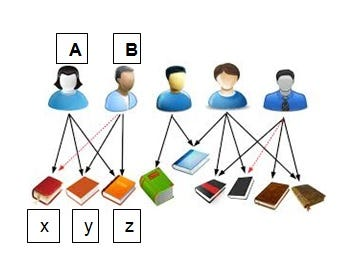Developing a Content Based Book Recommender System — Theory

Imagine you have a collection of data science books in your library and let’s say your friend has read a book on neural network and wants to read another book on the same topic to build up his/her knowledge on the subject. The best way is to implement a simple content based recommender system. We will look at three important concepts here which go into building this content based recommender system.
• Vectors
• TF-IDF
• Cosine Similarity
Vectors
The fundamental idea is to convert the texts or words into a vector and represent in a vector space model. This idea is so beautiful and in an essence this very idea of vectors is what is making the rapid strides in Machine learning and AI possible. In fact Geoffrey Hinton (“Father of Deep Learning”) in a MIT technology review article acknowledged that the AI institute at Toronto has been named “Vector Institute” owing to the beautiful properties of vectors that has helped them in the field of Deep Learning and other variants of Neural nets.

TF — IDF
TF- IDF stands for Term Frequency and Inverse Document Frequency .TF-IDF helps in evaluating importance of a word in a document.

TF — Term Frequency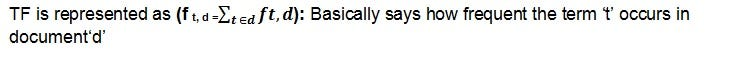In order to ascertain how frequent the term/word appears in the document and also to represent the document in vector form, let’s break it down to following steps.

Step 1:Create a dictionary of words (also known as bag of words) present in the whole document space. We ignore some common words also called as stop words e.g. the, of, a, an, is etc, since these words are pretty common and it will not help us in our goal of choosing important words
In this current example I have used the file ‘test1.csv’ which contains titles of 50 books. But to drive home the point, just consider 3 book titles (documents) to be making up the whole document space. So B1 is one document, B2 and B3 are other documents. Together B1, B2, B3 make up the document space.
B1 — Recommender Systems
B2 — The Elements of Statistical Learning
B3 — Recommender Systems — Advanced

Now creating an index of these words (stop words ignored)
1. Recommender 2. Systems 3 Elements 4. Statistical 5.Learning 6. Advanced

Step 2: Forming the vector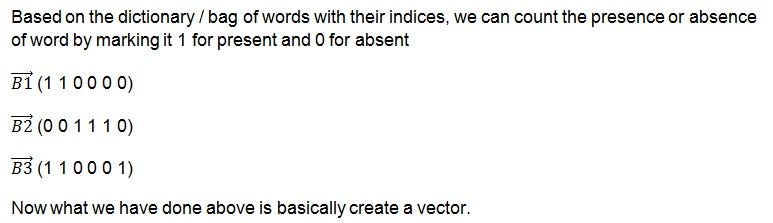The Term Frequency helps us identify how many times the term or word appears in a document but there is also an inherent problem, TF gives more importance to words/ terms occurring frequently while ignoring the importance of rare words/terms. This is not an ideal situation as rare words contain more importance or signal. This problem is resolved by IDF.
Sometimes a word / term might occur more frequently in longer documents than shorter ones; hence Term Frequency normalization is carried out.
TFn = (Number of times term t appears in a document) / (Total number of terms in the document), where n represents normalized.

IDF (Inverse Document Frequency):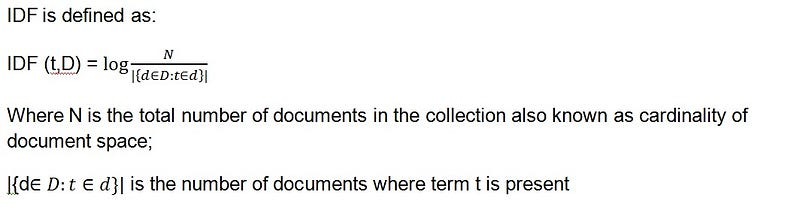In some variation of the IDF definition, 1 is added to the denominator so as to avoid a case of division by zero in the case of no terms being present in the document.
Basically a simple definition would be:
IDF = ln (Total number of documents / Number of documents with term t in it)
Now let’s take an example from our own dictionary or bag of words and calculate the IDFs We had 6 terms or words which are as follows
1. Recommender 2. Systems 3 Elements 4. Statistical 5.Learning 6. Advanced
and our documents were :
B1 — Recommender Systems
B2 — The Elements of Statistical Learning
B3 — Recommender Systems — Advanced
Now IDF (w1) = log 3/2; IDF(w2) = log 3/2; IDF (w3) = log 3/1; IDF (W4) = log 3/1; IDF (W5) = log 3/1; IDF(w6) = log 3/1
(note : natural logarithm being taken and w1..w6 denotes words/terms)

We then again get a vector as follows:
= (0.4054, 0.4054, 1.0986, 1.0986, 1.0986, 1.0986)

TF-IDF Weight:
Now the final step would be to get the TF-IDF weight. The TF vector and IDF vector are converted into a matrix. Then TF-IDF weight is represented as:
TF-IDF Weight = TF (t,d) * IDF(t,D)
This is the same matrix which we get by executing the below python code:
tfidf_matrix = tf.fit_transform(ds['Book Title'])

Cosine Similarity:
Well cosine similarity is a measure of similarity between two non zero vectors. One of the beautiful thing about vector representation is we can now see how closely related two sentence are based on what angles their respective vectors make.
Cosine value ranges from -1 to 1.
So if two vectors make an angle 0, then cosine value would be 1, which in turn would mean that the sentences are closely related to each other. If the two vectors are orthogonal, i.e. cos 90 then it would mean that the sentences are almost unrelated.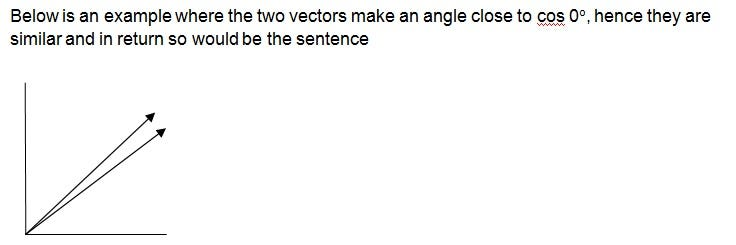Developing a Content Based Book Recommender System — Implementation

Below is the Gist link where I have written a few lines of code in python to implement a simple content based book recommender system. I have added comments (words after #) to make it clear what each line of code is doing.

```import pandas as pd from sklearn.feature_extraction.text import TfidfVectorizer from sklearn.metrics.pairwise import cosine_similarity ds = pd.read_csv("test1.csv") #you can plug in your own list of products or movies or books here as csv file# tf = TfidfVectorizer(analyzer='word', ngram_range=(1, 3), min_df=0, stop_words='english') #ngram explanation begins# #ngram (1,3) can be explained as follows# #ngram(1,3) encompasses uni gram, bi gram and tri gram #consider the sentence "The ball fell" #ngram (1,3) would be the, ball, fell, the ball, ball fell, the ball fell #ngram explanation ends# tfidf_matrix = tf.fit_transform(ds['Book Title']) cosine_similarities = cosine_similarity(tfidf_matrix,tfidf_matrix) results = {} # dictionary created to store the result in a dictionary format (ID : (Score,item_id))# for idx, row in ds.iterrows(): #iterates through all the rows # the below code 'similar_indice' stores similar ids based on cosine similarity. sorts them in ascending order. [:-5:-1] is then used so that the indices with most similarity are got. 0 means no similarity and 1 means perfect similarity# similar_indices = cosine_similarities[idx].argsort()[:-5:-1] #stores 5 most similar books, you can change it as per your needs similar_items = [(cosine_similarities[idx][i], ds['ID'][i]) for i in similar_indices] results[row['ID']] = similar_items[1:] #below code 'function item(id)' returns a row matching the id along with Book Title. Initially it is a dataframe, then we convert it to a list# def item(id): return ds.loc[ds['ID'] == id]['Book Title'].tolist() def recommend(id, num): if (num == 0): print("Unable to recommend any book as you have not chosen the number of book to be recommended") elif (num==1): print("Recommending " + str(num) + " book similar to " + item(id)) else : print("Recommending " + str(num) + " books similar to " + item(id)) print("----------------------------------------------------------") recs = results[id][:num] for rec in recs: print("You may also like to read: " + item(rec) + " (score:" + str(rec) + ")") #the first argument in the below function to be passed is the id of the book, second argument is the number of books you want to be recommended# recommend(5,2) ```

The Output:
``` Recommending 2 books similar to The Elements of Statistical Learning ---------------------------------------------------------- You may also like to read: An introduction to Statistical Learning (score:0.389869522721) You may also like to read: Statistical Distributions (score:0.13171009673) ```

Sources: Original. Reposted with permission.

Related:

## Top Stories Past 30 Days

Most PopularGet KDnuggets, a leading newsletter on AI, Data Science, and Machine Learning

By subscribing you accept KDnuggets Privacy Policy